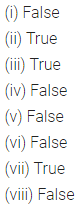ML Aggarwal Class 6 Solutions Chapter 11 Understanding Symmetrical Shapes Ex 11.4 for ICSE Understanding Mathematics acts as the best resource during your learning and helps you score well in your exams.

## ML Aggarwal Class 6 Solutions for ICSE Maths Chapter 11 Understanding Symmetrical Shapes Ex 11.4

Question 1.
Name the following triangles with regards to sides :Solution: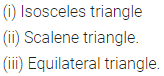Question 2.
Name the following triangles with regards to angles :Solution: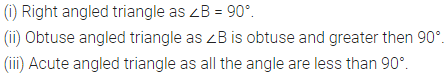Question 3.
Name each of the following triangles in two different ways (you may judge the nature of the angle by observation):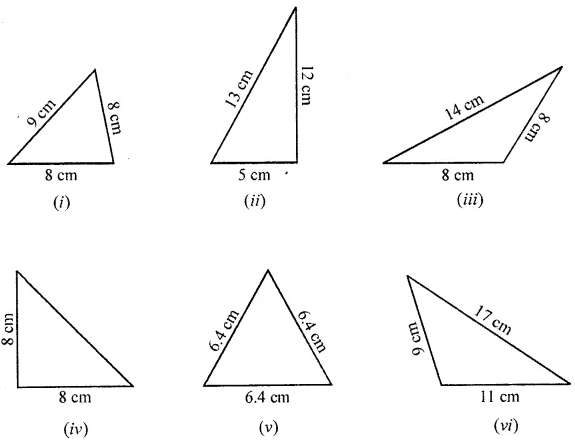Solution: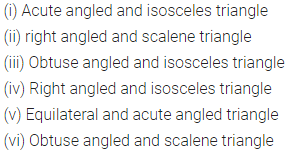Question 4.
Match the following: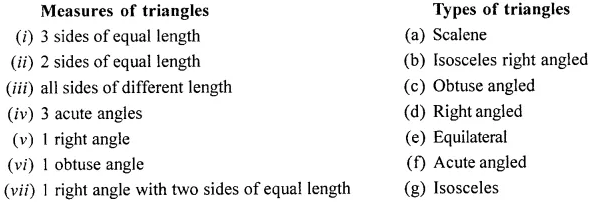Solution: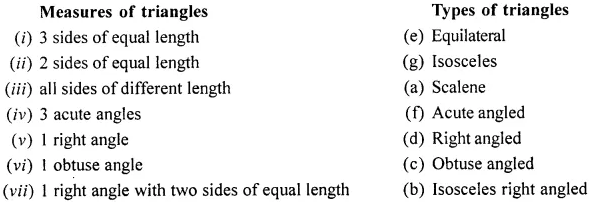Question 5.
State which of the following statement are true and which are false :
(i) A triangle can have two right angles.
(ii) A triangle cannot have more than one obtuse angle.
(iii) A triangle has atleast two actue angles.
(iv) If all the three sides of a triangle are equal, it is called a scalene triangle.
(v) A triangle has four sides.
(vi) An isosceles triangle is an equilateral triangle also.
(vii) An equilateral triangle is an isosceles triangle also.
(viii)An scalene triangle has all its angles equal.
Solution: